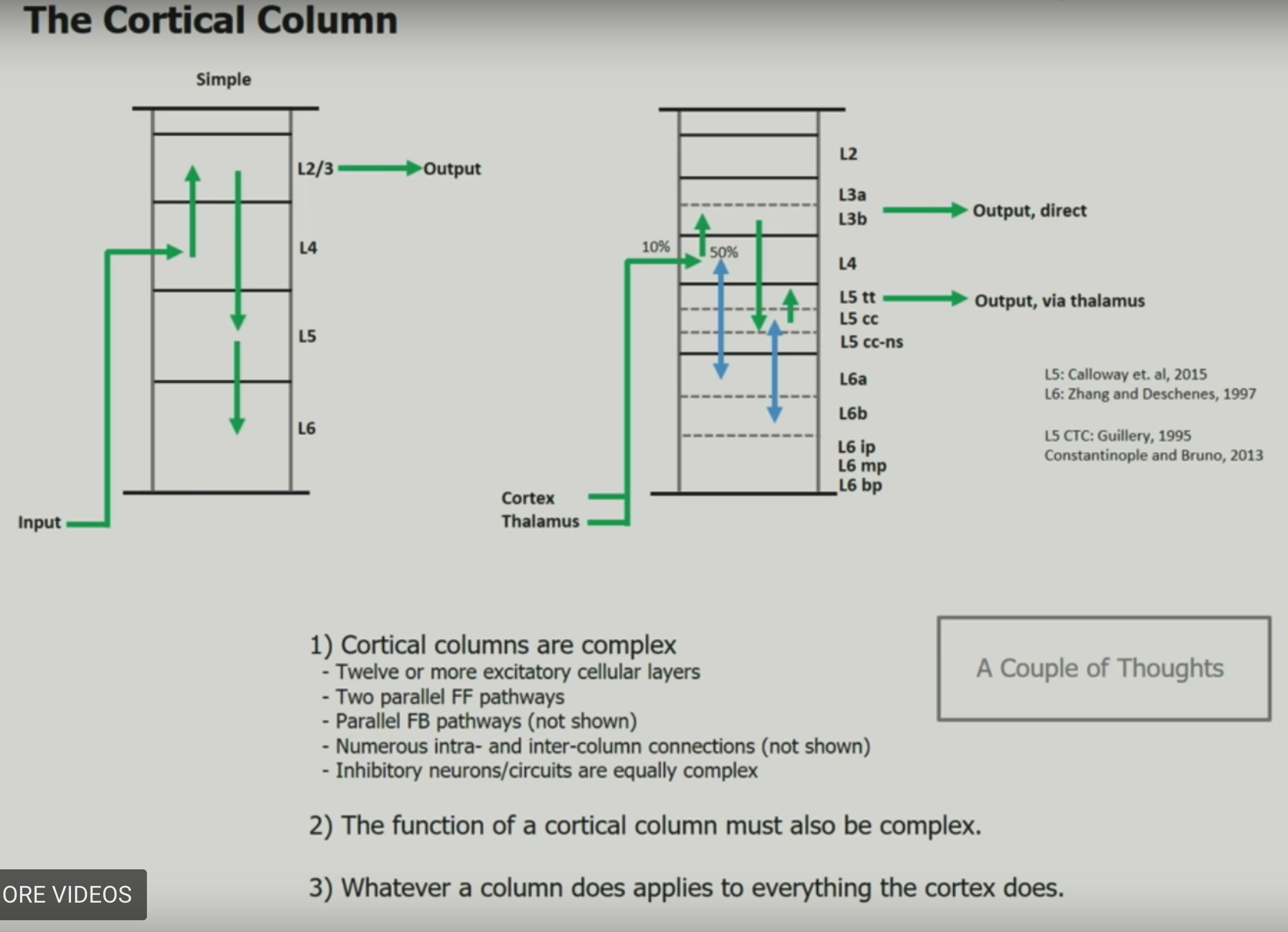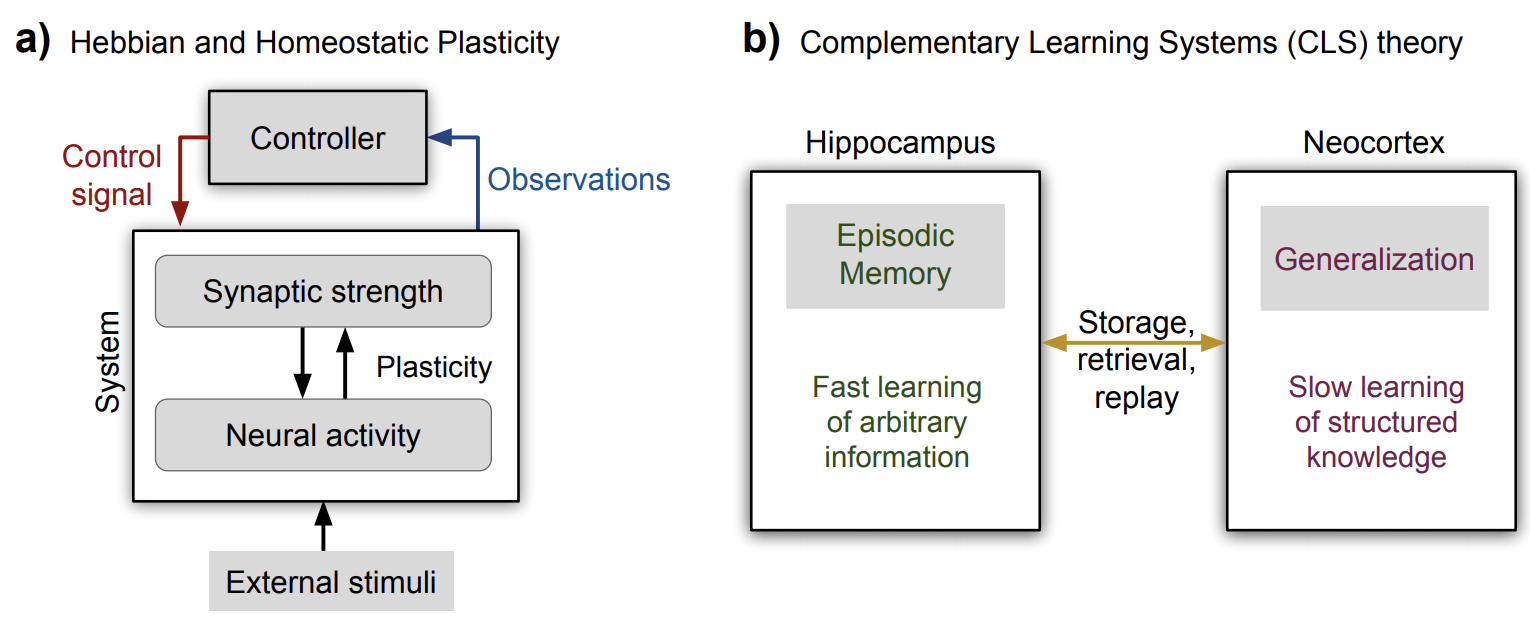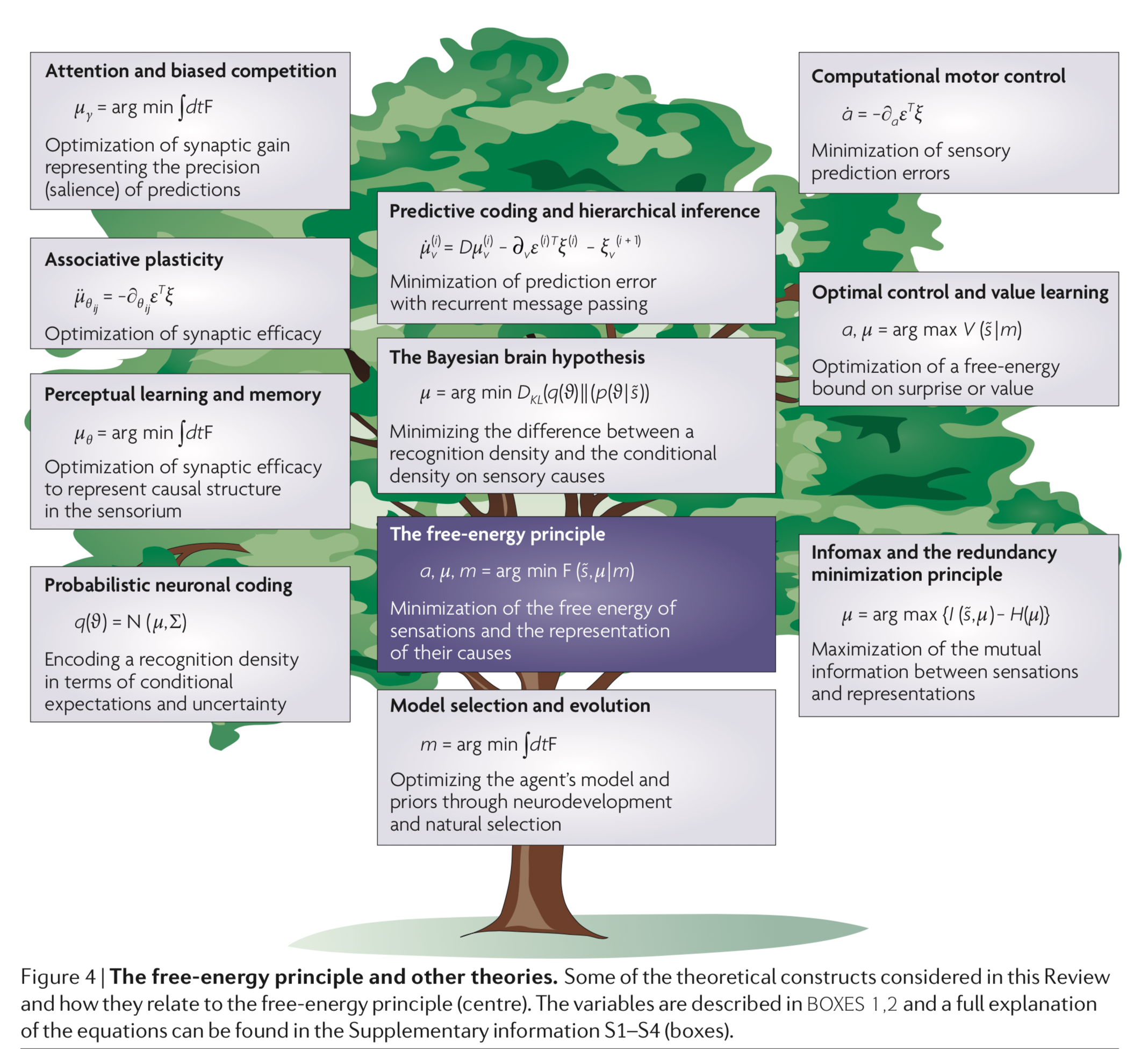# 1.1. comp neuro¶

## 1.1.2. high-dimensional computing¶

• high-level overview

• current inspiration has all come from single neurons at a time - hd computing is going past this

• the brain’s circuits are high-dimensional

• elements are stochastic not deterministic

• can learn from experience

• no 2 brains are alike yet they exhibit the same behavior

• basic question of comp neuro: what kind of computing can explain behavior produced by spike trains?

• recognizing ppl by how they look, sound, or behave

• learning from examples

• remembering things going back to childhood

• communicating with language

• HD computing overview paper

• in these high dimensions, most points are close to equidistant from one another (L1 distance), and are approximately orthogonal (dot product is 0)

• memory

• heteroassociative - can return stored X based on its address A

• autoassociative - can return stored X based on a noisy version of X (since it is a point attractor), maybe with some iteration

• this adds robustness to the memory

• this also removes the need for addresses altogether

### 1.1.2.1. definitions¶

• what is hd computing?

• compute with random high-dim vectors

• ex. 10k vectors A, B of +1/-1 (also extends to real / complex vectors)

• 3 operations

• addition: A + B = (0, 0, 2, 0, 2,-2, 0, ….)

• multiplication: A * B = (-1, -1, -1, 1, 1, -1, 1, …) - this is XOR

• want this to be invertible, dsitribute over addition, preserve distance, and be dissimilar to the vectors being multiplied

• number of ones after multiplication is the distance between the two original vectors

• can represent a dissimilar set vector by using multiplication

• permutation: shuffles values

• ex. rotate (bit shift with wrapping around)

• multiply by rotation matrix (where each row and col contain exactly one 1)

• can think of permutation as a list of numbers 1, 2, …, n in permuted order

• many properties similar to multiplication

• random permutation randomizes

• basic operations

• weighting by a scalar

• similarity = dot product (sometimes normalized)

• A $$\cdot$$ A = 10k

• A $$\cdot$$ A = 0 (orthogonal)

• in high-dim spaces, almost all pairs of vectors are dissimilar A $$\cdot$$ B = 0

• goal: similar meanings should have large similarity

• normalization

• for binary vectors, just take the sign

• for non-binary vectors, scalar weight

• data structures

• these operations allow for encoding all normal data structures: sets, sequences, lists, databases

• set - can represent with a sum (since the sum is similar to all the vectors)

• can find a stored set using any element

• if we don’t store the sum, can probe with the sum and keep subtracting the vectors we find

• multiset = bag (stores set with frequency counts) - can store things with order by adding them multiple times, but hard to actually retrieve frequencies

• sequence - could have each element be an address pointing to the next element

• problem - hard to represent sequences that share a subsequence (could have pointers which skip over the subsquence)

• soln: index elements based on permuted sums

• can look up an element based on previous element or previous string of elements

• could do some kind of weighting also

• pairs - could just multiply (XOR), but then get some weird things, e.g. A * A = 0

• instead, permute then multiply

• can use these to index (address, value) pairs and make more complex data structures

• named tuples - have smth like (name: x, date: m, age: y) and store as holistic vector $$H = N*X + D * M + A * Y$$

• individual attribute value can be retrieved using vector for individual key

• representation substituting is a little trickier….

• we blur what is a value and whit is a variable

• can do this for a pair or for a named tuple with new values

• this doesn’t always work

• examples

• context vectors

• standard practice (e.g. LSA): make matrix of word counts, where each row is a word, and each column is a document

• HD computing alternative: each row is a word, but each document is assigned a few ~10 columns at random

• thus, the number of columns doesn’t scale with the number of documents

• can also do this randomness for the rows (so the number of rows < the number of words)

• can still get semantic vector for a row/column by adding together the rows/columns which are activated by that row/column

• this examples still only uses bag-of-words (but can be extended to more)

• learning rules by example

• particular instance of a rule is a rule (e.g mother-son-baby $$\to$$ grandmother)

• as we get more examples and average them, the rule gets better

• doesn’t always work (especially when things collapse to identity rule)

• analogies from pairs

• ex. what is the dollar of mexico?

### 1.1.2.2. ex. identify the language¶

• paper: LANGUAGE RECOGNITION USING RANDOM INDEXING (joshi et al. 2015)

• benefits - very simple and scalable - only go through data once

• equally easy to use 4-grams vs. 5-grams

• data

• train: given million bytes of text per language (in the same alphabet)

• test: new sentences for each language

• training: compute a 10k profile vector for each language and for each test sentence

• could encode each letter wih a seed vector which is 10k

• instead encode trigrams with rotate and multiply

• 1st letter vec rotated by 2 * 2nd letter vec rotated by 1 * 3rd letter vec

• ex. THE = r(r(T)) * r(H) * r(E)

• approximately orthogonal to all the letter vectors and all the other possible trigram vectors…

• profile = sum of all trigram vectors (taken sliding)

• ex. banana = ban + ana + nan + ana

• profile is like a histogram of trigrams

• testing

• compare each test sentence to profiles via dot product

• clusters similar languages - cool!

• gets 97% test acc

• can query the letter most likely to follor “TH”

• form query vector $$Q = r(r(T)) * r(H)$$

• query by using multiply X + Q * english-profile-vec

• find closest letter vecs to X - yields “e”

### 1.1.2.3. details¶

• mathematical background

• randomly chosen vecs are dissimilar

• sum vector is similar to its argument vectors

• product vector and permuted vector are dissimilar to their argument vectors

• multiplication distibutes over addition

• permutation distributes over both additions and multiplication

• multiplication and permutations are invertible

• addition is approximately invertible

• comparison to DNNs

• both do statistical learning from data

• data can be noisy

• both use high-dim vecs although DNNs get bad with him dims (e.g. 100k)

• HD is founded on rich mathematical theory

• new codewords are made from existing ones

• HD memory is a separate func

• HD algos are transparent, incremental (on-line), scalable

• somewhat closer to the brain…cerebellum anatomy seems to be match HD

• HD: holistic (distributed repr.) is robust

• different names

• Tony plate: holographic reduced representation

• ross gayler: multiply-add-permute arch

• gayler & levi: vector-symbolic arch

• gallant & okaywe: matrix binding with additive termps

• fourier holographic reduced reprsentations (FHRR; Plate)

• …many more names

• theory of sequence indexing and working memory in RNNs

• trying to make key-value pairs

• VSA as a structured approach for understanding neural networks

• reservoir computing = state-dependent network = echos-state network = liquid state machine - try to represen sequential temporal data - builds representations on the fly

## 1.1.3. dnns with memory¶

• Neural Statistician (Edwards & Storkey, 2016) summarises a dataset by averaging over their embeddings

• kanerva machine

• like a VAE where the prior is derived from an adaptive memory store

## 1.1.5. dynamic routing between capsules¶

• hinton 1981 - reference frames requires structured representations

• mapping units vote for different orientations, sizes, positions based on basic units

• mapping units gate the activity from other types of units - weight is dependent on if mapping is activated

• top-down activations give info back to mapping units

• this is a hopfield net with three-way connections (between input units, output units, mapping units)

• reference frame is a key part of how we see - need to vote for transformations

• olshausen, anderson, & van essen 1993 - dynamic routing circuits

• ran simulations of such things (hinton said it was hard to get simulations to work)

• we learn things in object-based reference frames

• inputs -> outputs has weight matrix gated by control

• zeiler & fergus 2013 - visualizing things at intermediate layers - deconv (by dynamic routing)

• save indexes of max pooling (these would be the control neurons)

• when you do deconv, assign max value to these indexes

• arathom 02 - map-seeking circuits

• tenenbaum & freeman 2000 - bilinear models

• trying to separate content + style

• hinton et al 2011 - transforming autoencoders - trained neural net to learn to shift imge

• sabour et al 2017 - dynamic routing between capsules

• units output a vector (represents info about reference frame)

• matrix transforms reference frames between units

• recurrent control units settle on some transformation to identify reference frame

• notes from this blog post

• problems with cnns

• pooling loses info

• don’t account for spatial relations between image parts

• can’t transfer info to new viewpoints

• capsule - vector specifying the features of an object (e.g. position, size, orientation, hue texture) and its likelihood

• ex. an “eye” capsule could specify the probability it exists, its position, and its size

• magnitude (i.e. length) of vector represents probability it exists (e.g. there is an eye)

• direction of vector represents the instatntiation parameters (e.g. position, size)

• hierarchy

• capsules in later layers are functions of the capsules in lower layers, and since capsule has extra properties can ask questions like “are both eyes similarly sized?”

• equivariance = we can ensure our net is invariant to viewpoints by checking for all similar rotations/transformations in the same amount/direction

• active capsules at one level make predictions for the instantiation parameters of higher-level capsules

• when multiple predictions agree, a higher-level capsule is activated

• steps in a capsule (e.g. one that recognizes faces)

• receives an input vector (e.g. representing eye)

• apply affine transformation - encodes spatial relationships (e.g. between eye and where the face should be)

• applying weighted sum by the C weights, learned by the routing algorithm

• these weights are learned to group similar outputs to make higher-level capsules

• vectors are squashed so their magnitudes are between 0 and 1

• outputs a vector

## 1.1.6. hierarchical temporal memory (htm)¶

• binary synapses and learns by modeling the growth of new synapses and the decay of unused synapses

• separate aspects of brains and neurons that are essential for intelligence from those that depend on brain implementation

### 1.1.6.1. necortical structure¶

• evolution leads to physical/logical hierarchy of brain regions

• neocortex is like a flat sheet

• neocortex regions are similar and do similar computation

• Mountcastle 1978: vision regions are vision becase they receive visual input

• number of regions / connectivity seems to be genetic

• before necortex, brain regions were homogenous: spinal cord, brain stem, basal ganglia, …

•### 1.1.6.2. principles¶

• common algorithims accross neocortex

• hierarchy

• sparse distributed representations (SDR) - vectors with thousands of bits, mostly 0s

• bits of representation encode semantic properties

• inputs

• data from the sense

• copy of the motor commands

• “sensory-motor” integration - perception is stable while the eyes move

• patterns are constantly changing

• necortex tries to control old brain regions which control muscles

• learning: region accepts stream of sensory data + motor commands

• learns of changes in inputs

• ouputs motor commands

• only knows how its output changes its input

• must learn how to control behavior via associative linking

• sensory encoders - takes input and turnes it into an SDR

• engineered systems can use non-human senses

• behavior needs to be incorporated fully

• temporal memory - is a memory of sequences

• everything the neocortex does is based on memory and recall of sequences of patterns

• on-line learning

• prediction is compared to what actually happens and forms the basis of learning

• minimize the error of predictions

### 1.1.6.3. papers¶

• “A Theory of How Columns in the Neocortex Enable Learning the Structure of the World”

• network model that learns the structure of objects through movement

• object recognition

• over time individual columns integrate changing inputs to recognize complete objects

• through existing lateral connections

• within each column, neocortex is calculating a location representation

• locations relative to each other = allocentric

• much more motion involved

• multiple columns - integrate spatial inputs - make things fast

• single column - integrate touches over time - represent objects properly

• “Why Neurons Have Thousands of Synapses, A Theory of Sequence Memory in Neocortex”

• learning and recalling sequences of patterns

• neuron with lots of synapses can learn transitions of patterns

• network of these can form robust memory

## 1.1.7. forgetting¶

• Continual Lifelong Learning with Neural Networks: A Review

• main issues is catastrophic forgetting / stability-plasticity dilemma

•• 2 types of plasticity

• Hebbian plasticity (Hebb 1949) for positive feedback instability

• compensatory homeostatic plasticity which stabilizes neural activity

• approaches: regularization, dynamic architectures (e.g. add more nodes after each task), memory replay

## 1.1.8. deeptune-style¶

• ponce_19_evolving_stimuli: https://www.cell.com/action/showPdf?pii=S0092-8674%2819%2930391-5

• bashivan_18_ann_synthesis

• adept paper

• use kernel regression from CNN embedding to calculate distances between preset images

• select preset images

• verified with macaque v4 recording

• currently only study that optimizes firing rates of multiple neurons

• pick next stimulus in closed-loop (“adaptive sampling” = “optimal experimental design”)

• J. Benda, T. Gollisch, C. K. Machens, and A. V. Herz, “From response to stimulus: adaptive sampling in sensory physiology”

• find the smallest number of stimuli needed to fit parameters of a model that predicts the recorded neuron’s activity from the stimulus

• maximizing firing rates via genetic algorithms

• maximizing firing rate via gradient ascent

• C. DiMattina and K. Zhang,“Adaptive stimulus optimization for sensory systems neuroscience”](https://www.frontiersin.org/articles/10.3389/fncir.2013.00101/full)

• 2 general approaches: gradient-based approaches + genetic algorithms

• can put constraints on stimulus space

• stimulus adaptation

• might want iso-response surfaces

• maximally informative stimulus ensembles (Machens, 2002)

• model-fitting: pick to maximize info-gain w/ model params

• using fixed stimulus sets like white noise may be deeply problematic for efforts to identify non-linear hierarchical network models due to continuous parameter confounding (DiMattina and Zhang, 2010)

• use for model selection

## 1.1.9. population coding¶

• saxena_19_pop_cunningham: “Towards the neural population doctrine”

• correlated trial-to-trial variability

• Ni et al. showed that the correlated variability in V4 neurons during attention and learning — processes that have inherently different timescales — robustly decreases

• ‘choice’ decoder built on neural activity in the first PC performs as well as one built on the full dataset, suggesting that the relationship of neural variability to behavior lies in a relatively small subspace of the state space.

• decoding

• more neurons only helps if neuron doesn’t lie in span of previous neurons

• encoding

• can train dnn goal-driven or train dnn on the neural responses directly

• testing

• important to be able to test population structure directly

• population vector coding - ex. neurons coded for direction sum to get final direction

• reduces uncertainty

• correlation coding - correlations betweeen spikes carries extra info

• independent-spike coding - each spike is independent of other spikes within the spike train

• position coding - want to represent a position

• for grid cells, very efficient

• sparse coding

• hard when noise between neurons is correlated

• measures of information

• eda

• plot neuron responses

• calc neuron covariances

## 1.1.10. interesting misc papers¶

• berardino 17 eigendistortions

• Fisher info matrix under certain assumptions = $$Jacob^TJacob$$ (pixels x pixels) where Jacob is the Jacobian matrix for the function f action on the pixels x

• most and least noticeable distortion directions corresponding to the eigenvectors of the Fisher info matrix

• gao_19_v1_repr

• don’t learn from images - v1 repr should come from motion like it does in the real world

• repr

• vector of local content

• matrix of local displacement

• why is this repr nice?

• separate reps of static image content and change due to motion

• disentangled rotations

• learning

• predict next image given current image + displacement field

• predict next image vector given current frame vectors + displacement

• kietzmann_18_dnn_in_neuro_rvw

• friston_10_free_energy

•